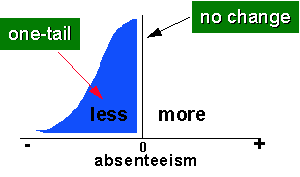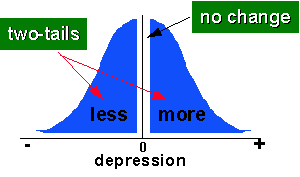Hypotheses

# Hypotheses

An hypothesis is a specific statement of prediction. It describes in concrete (rather than theoretical) terms what you expect will happen in your study. Not all studies have hypotheses. Sometimes a study is designed to be exploratory (see inductive research). There is no formal hypothesis, and perhaps the purpose of the study is to explore some area more thoroughly in order to develop some specific hypothesis or prediction that can be tested in future research. A single study may have one or many hypotheses.

Actually, whenever I talk about an hypothesis, I am really thinking simultaneously about two hypotheses. Let’s say that you predict that there will be a relationship between two variables in your study. The way we would formally set up the hypothesis test is to formulate two hypothesis statements, one that describes your prediction and one that describes all the other possible outcomes with respect to the hypothesized relationship. Your prediction is that variable `A` and variable `B` will be related (you don’t care whether it’s a positive or negative relationship). Then the only other possible outcome would be that variable `A` and variable `B` are not related. Usually, we call the hypothesis that you support (your prediction) the alternative hypothesis, and we call the hypothesis that describes the remaining possible outcomes the null hypothesis. Sometimes we use a notation like `HA` or `H1` to represent the alternative hypothesis or your prediction, and `HO` or `H0` to represent the null case. You have to be careful here, though. In some studies, your prediction might very well be that there will be no difference or change. In this case, you are essentially trying to find support for the null hypothesis and you are opposed to the alternative.

If your prediction specifies a direction, and the null therefore is the no difference prediction and the prediction of the opposite direction, we call this a one-tailed hypothesis. For instance, let’s imagine that you are investigating the effects of a new employee training program and that you believe one of the outcomes will be that there will be less employee absenteeism. Your two hypotheses might be stated something like this:

The null hypothesis for this study is:

HO: As a result of the XYZ company employee training program, there will either be no significant difference in employee absenteeism or there will be a significant increase.

which is tested against the alternative hypothesis:

HA: As a result of the XYZ company employee training program, there will be a significant decrease in employee absenteeism.In the figure on the left, we see this situation illustrated graphically. The alternative hypothesis – your prediction that the program will decrease absenteeism – is shown there. The null must account for the other two possible conditions: no difference, or an increase in absenteeism. The figure shows a hypothetical distribution of absenteeism differences. We can see that the term “one-tailed” refers to the tail of the distribution on the outcome variable.

When your prediction does not specify a direction, we say you have a two-tailed hypothesis. For instance, let’s assume you are studying a new drug treatment for depression. The drug has gone through some initial animal trials, but has not yet been tested on humans. You believe (based on theory and the previous research) that the drug will have an effect, but you are not confident enough to hypothesize a direction and say the drug will reduce depression (after all, you’ve seen more than enough promising drug treatments come along that eventually were shown to have severe side effects that actually worsened symptoms). In this case, you might state the two hypotheses like this:

The null hypothesis for this study is:

HO: As a result of 300mg./day of the ABC drug, there will be no significant difference in depression.

which is tested against the alternative hypothesis:

HA: As a result of 300mg./day of the ABC drug, there will be a significant difference in depression.The figure on the right illustrates this two-tailed prediction for this case. Again, notice that the term “two-tailed” refers to the tails of the distribution for your outcome variable.

The important thing to remember about stating hypotheses is that you formulate your prediction (directional or not), and then you formulate a second hypothesis that is mutually exclusive of the first and incorporates all possible alternative outcomes for that case. When your study analysis is completed, the idea is that you will have to choose between the two hypotheses. If your prediction was correct, then you would (usually) reject the null hypothesis and accept the alternative. If your original prediction was not supported in the data, then you will accept the null hypothesis and reject the alternative. The logic of hypothesis testing is based on these two basic principles:

• the formulation of two mutually exclusive hypothesis statements that, together, exhaust all possible outcomes
• the testing of these so that one is necessarily accepted and the other rejected

OK, I know it’s a convoluted, awkward and formalistic way to ask research questions. But it encompasses a long tradition in statistics called the hypothetical-deductive model, and sometimes we just have to do things because they’re traditions. And anyway, if all of this hypothesis testing was easy enough so anybody could understand it, how do you think statisticians would stay employed?